# HMM 模型以及维特比(Viterbi) 算法 | 浅书 - AI学习笔记

## 马尔可夫链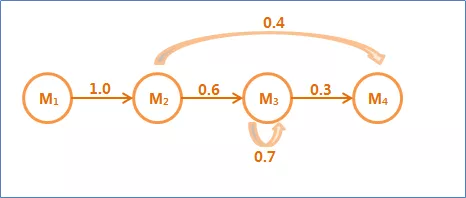## 隐含马尔可夫模型（HMM）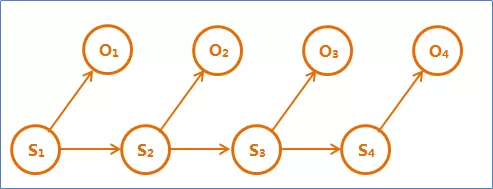HMM是一个五元组（O , Q , O0，A , B）:

1、O:{o1,o2,…,ot}是状态集合，也称为观测序列。

2、Q:{q1,q2,…,qv}是一组输出结果，也称为隐序列。

3、Aij = P(qj|qi)：转移概率分布

4、Bij = P(oj|qi)：发射概率分布

5、O0是初始状态，有些还有终止状态。

## HMM模型+维特比算法实例

### 2、已知信息

Healthy: 0.6;
Fever: 0.4;

Healthy -> normal: 0.5,
Healthy -> cold: 0.4,
Healthy -> dizzy: 0.1,
Fever -> normal: 0.1,
Fever -> cold: 0.3,
Fever -> dizzy: 0.6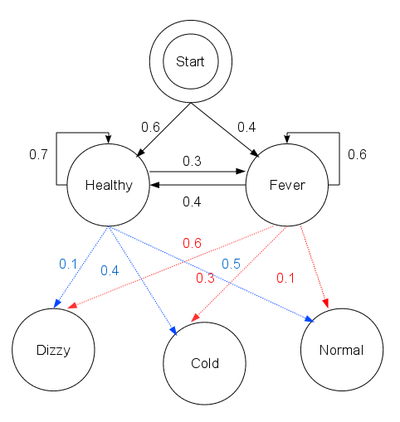### 3、求解

• δ1( H ) = p( H ) * p( N | H ) = 0.5 * 0.6 = 0.3
• δ1( F ) = p( F ) * p( N | F ) = 0.1 * 0.4 = 0.04

p( H -> H ) = 0.7
p( H -> F ) = 0.3
p( F -> H ) = 0.4
p( F -> F ) = 0.6

• δ2( H ) = Max[ δ1( H ) * p( H -> H ), δ1( F ) * p( F -> H ) ] * 0.4 = Max[0.21, 0.016] * 0.4 = 0.084
• δ2( F ) = Max[ δ1( H ) * p( H -> F ), δ1( F ) * p( F -> F ) ] * 0.3 = Max[0.09, 0.024] * 0.3 = 0.027

• δ3( H ) = Max[ δ2( H ) * p( H -> H ), δ2( F ) * p( F -> H ) ] * 0.4 = 0.00588
• δ3( F ) = Max[ δ2( H ) * p( H -> F ), δ2( F ) * p( F -> F ) ] * 0.3 = Max[0.09, 0.024] * 0.3 = 0.01512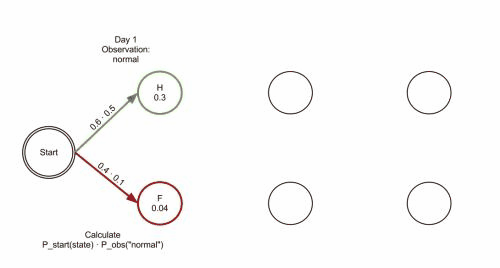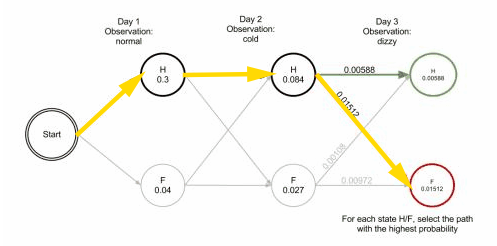## 代码实现

```# 状态的样本空间
states = ('Healthy', 'Fever')
# 观测的样本空间
observations = ('normal', 'cold', 'dizzy')
# 起始个状态概率
start_probability = {'Healthy': 0.6, 'Fever': 0.4}
# 状态转移概率
transition_probability = {
'Healthy' : {'Healthy': 0.7, 'Fever': 0.3},
'Fever' : {'Healthy': 0.4, 'Fever': 0.6},
}
# 状态->观测的发散概率
emission_probability = {
'Healthy' : {'normal': 0.5, 'cold': 0.4, 'dizzy': 0.1},
'Fever' : {'normal': 0.1, 'cold': 0.3, 'dizzy': 0.6},
}
​
​
def viterbi(obs, states, start_p, trans_p, emit_p):
# V 中第 t 个元素保存到时间t时，状态为Healthy和Fever的概率
V = [{}]
# path 保存 V 中各个时间概率最大的 state 所构成的路径
# 保存前一时t-1刻所有节点中到t时刻某节点的最优值的路径
path = {}
​
# 初始化
# 计算 δ1( H ) 和 δ1( F )
# 得到 [{'Healthy': 0.3, 'Fever': 0.04000000000000001}]
# emit_p 为状态->观测的发散概率
for y in states:
V[y] = start_p[y] * emit_p[y][obs]
path[y] = [y]
​
# Run Viterbi for t > 0
for t in range(1,len(obs)):
V.append({})
newpath = {}
​
for y in states:
# y 表示当前状态（先是 Healthy, 后是 Fever）
# prob: 前一天 (t - 1时) 所有状态转移为 y 时的最大的概率
# state: 最大概率时，对应的前一时刻t - 1的状态 y0
(prob, state) = max([ (V[t-1][y0] * trans_p[y0][y] * emit_p[y][obs[t]], y0) for y0 in states])
# 在 V 的时刻 t 中保存转换为状态y的最大概率值
V[t][y] = prob
# 得到现在状态为 state 的概率比较大，于是保留t-1时刻 path 中状态为 state 所经过的节点
newpath[y] = path[state] + [y]
​
# 修改 path 为最新得到的newpath
path = newpath
​
# prob: 当前时刻 t 所有状态对应的概率最大的概率
# state: prob对应的状态
(prob, state) = max([(V[len(obs) - 1][y], y) for y in states])
return (prob, path[state])
​
def example():
return viterbi(observations,
states,
start_probability,
transition_probability,
emission_probability)
print(example())
# 输出(0.01512, ['Healthy', 'Healthy', 'Fever'])
# 表示隐含序列为：'Healthy', 'Healthy', 'Fever'
```

## 参考链接

https://www.zhihu.com/question/20136144
https://www.cnblogs.com/Denise-hzf/p/6612212.html
https://zh.wikipedia.org/wiki/%E7%BB%B4%E7%89%B9%E6%AF%94%E7%AE%97%E6%B3%95